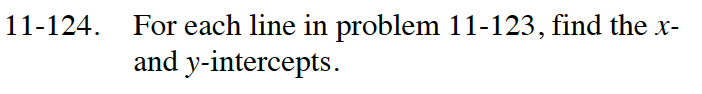### Home > CC4 > Chapter 11 > Lesson 11.2.6 > Problem11-124

11-124.

For each line in problem 11‑123, find the x‑ and y‑intercepts. Homework Help ✎The y-intercepts are shown on the graph.
Let y = 0 in the equations and solve to find the x-intercepts.

Top Line: y-intercept: (0, 10)
x-intercept: (40, 0)
Bottom Line: y-intercept: (0, 3)
x-intercept: (−9, 0)